Conge 精进

# AI 笔记 Week 10 Machine Learning

2017-12-09

This week you should finish Lesson 7, Machine Learning, and read Chapter 18.6-11 & 20.3 in Russell & Norvig.

Assignment 4: Decision Trees Due: October 29 at 11:59PM UTC-12 (Anywhere on Earth time)

# Boosting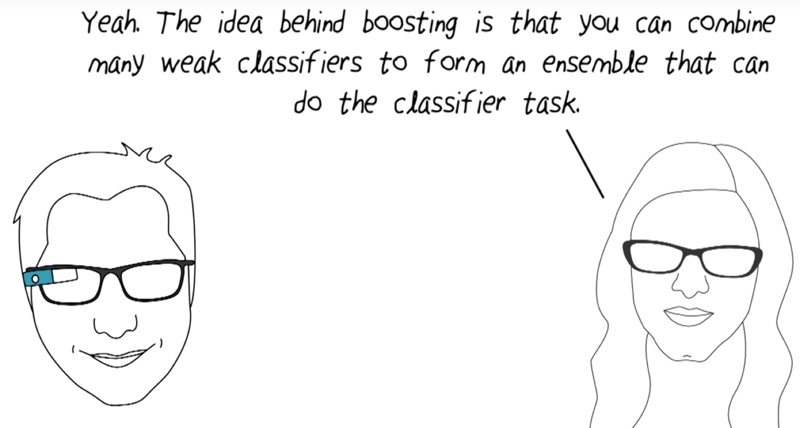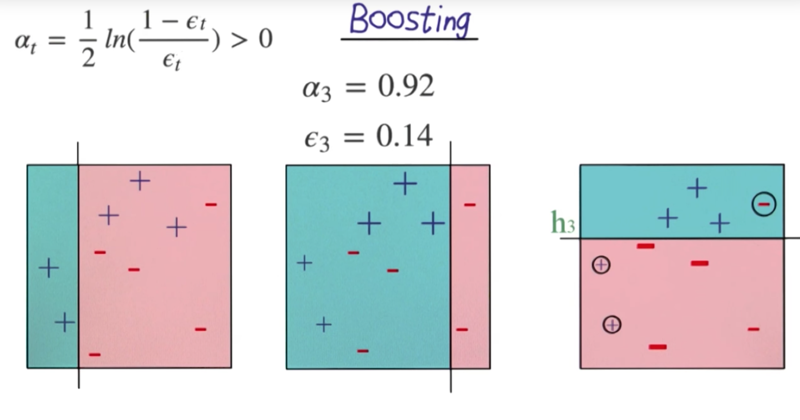• ??? I don’t know how to calculate e2 and e3???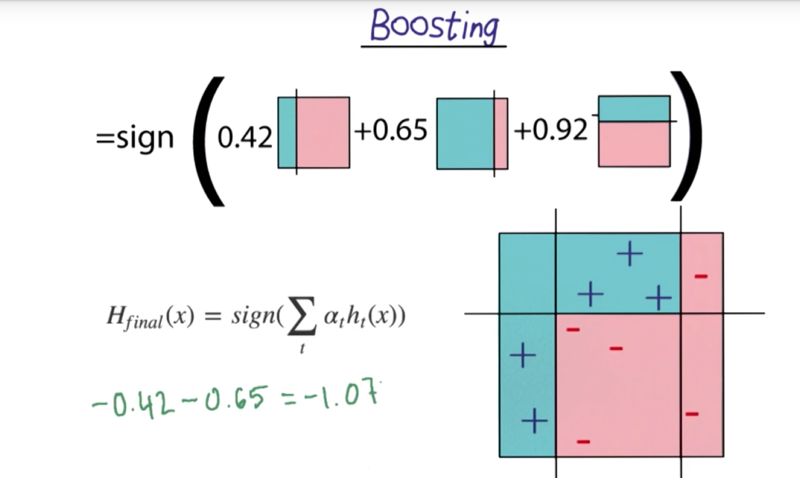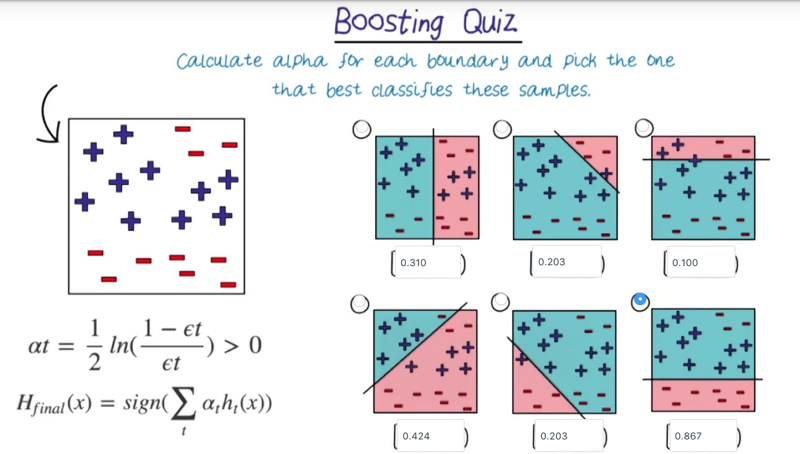# Neural nets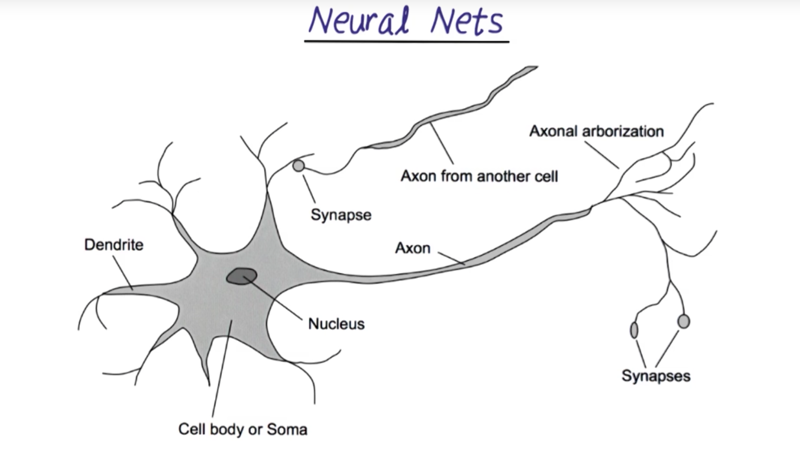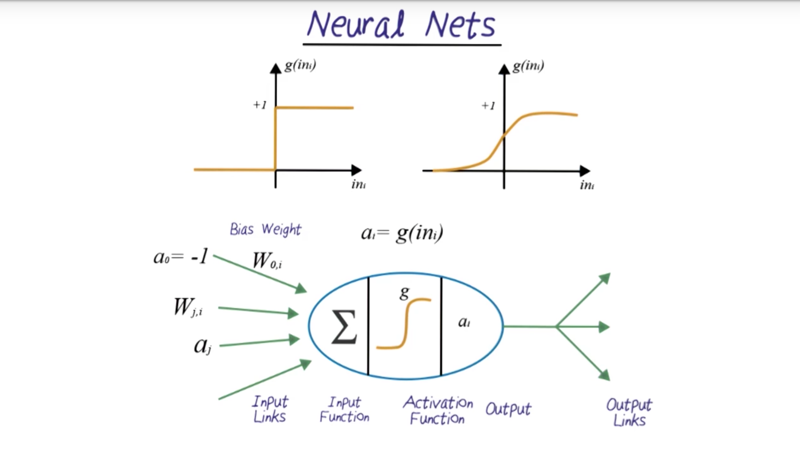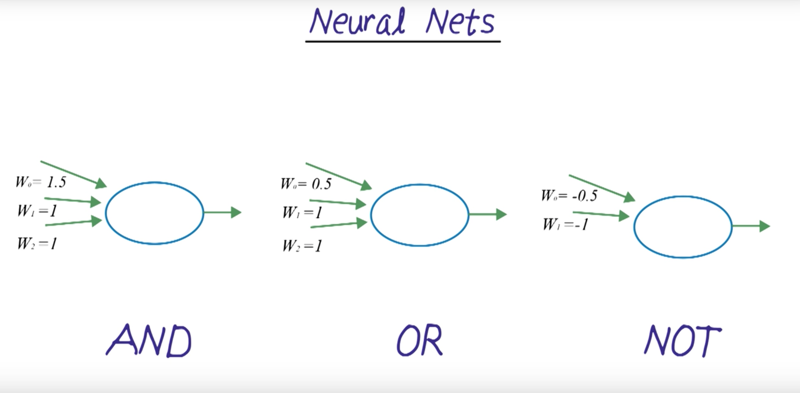Quiz: Neural Nets Quiz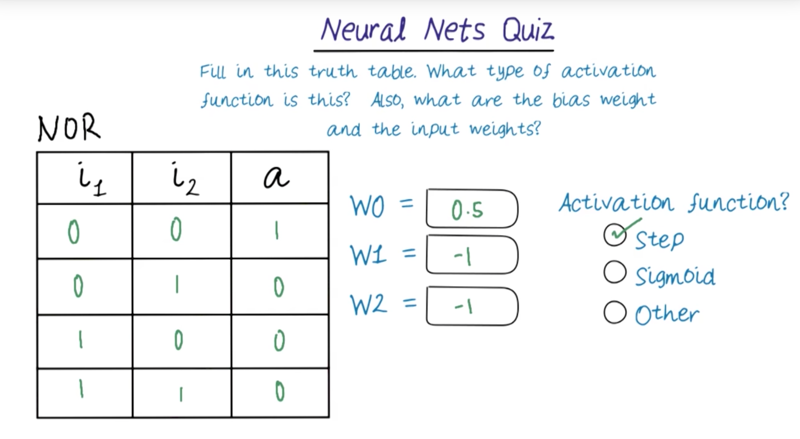Fill in the truth table for NOR and find weights such that:

a = { true if w0 + i1 w1 + i2 w2 > 0, else false }

Truth table Enter 1 for True, and 0 (or leave blank) for False in each cell. All combinations of i1 and i2 must be specified. Weights Each weight must be a number between 0.0 and 1.0, accurate to one or two decimal places. w1 and w2 are the input weights corresponding to i1 and i2 respectively. w0 is the bias weight. Activation function Choose the simplest activation function that can be used to capture this relationship.

## Multilayer Nets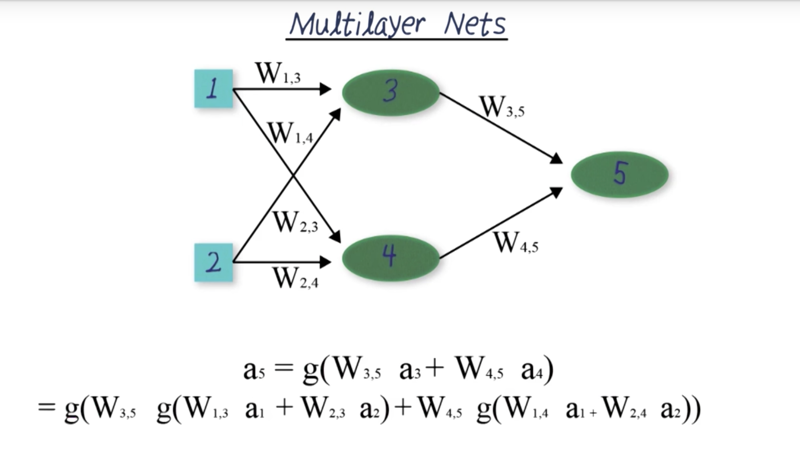• neural nets only makes sense when activation functions are nonlinear. If they are linear, the who network can be reduced to a linear function thus lose the power of the network.

## Perceptron Learning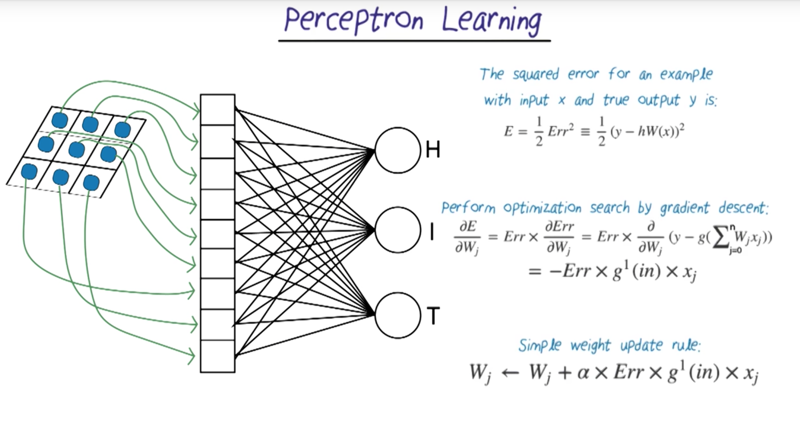• single layer perceptron can only generate linear boundaries.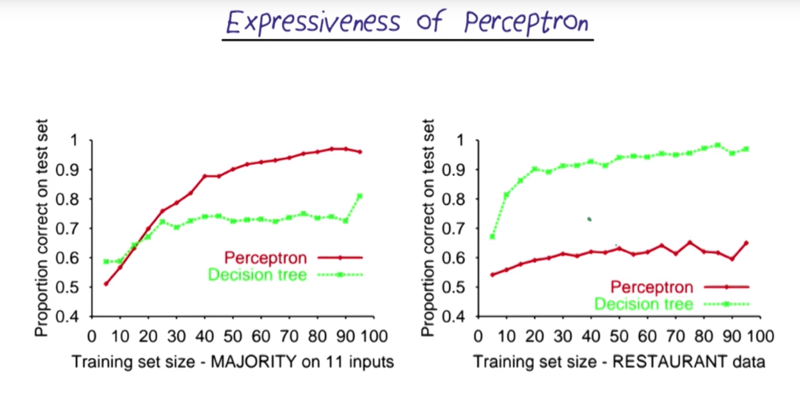• the performance of perceptrons is not always better than other methods (e.g. decision tree). it can be improved, however, by adding more layers

## Multilayer Perceptrons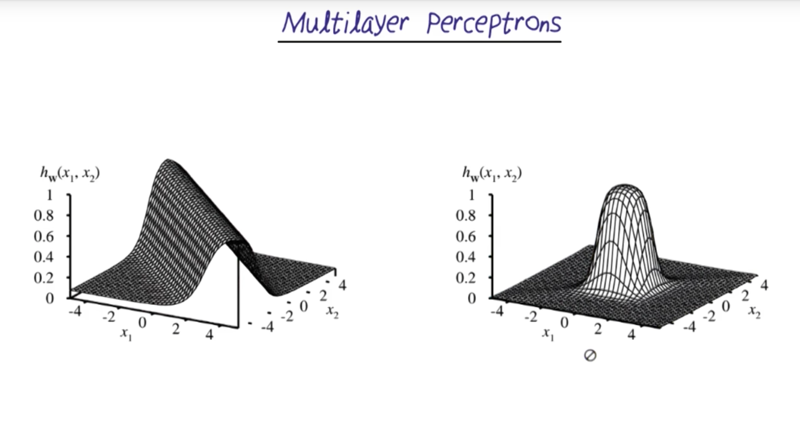## Back-Propagation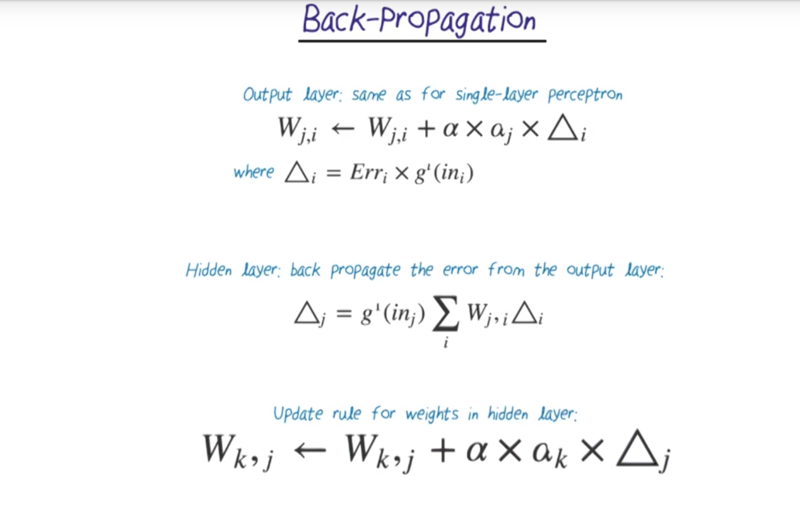• Back-Propagation is the way to calculate neural nets.
• the harder a problem is, the longer time for the algorithm to converge. Below are examples how fast an algorithm converges.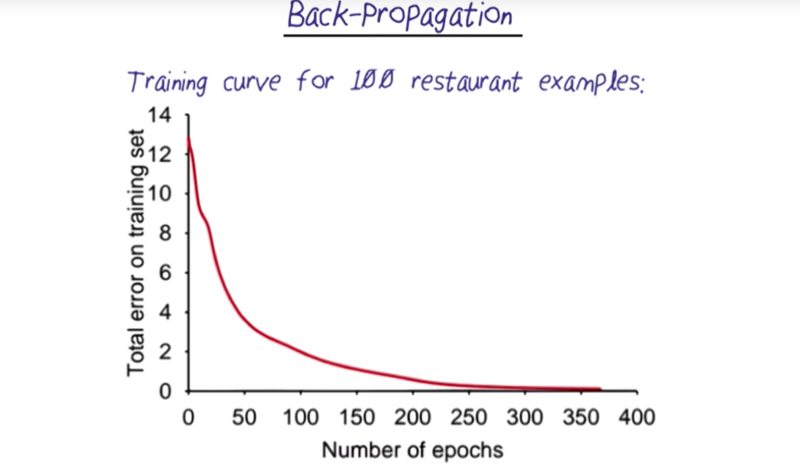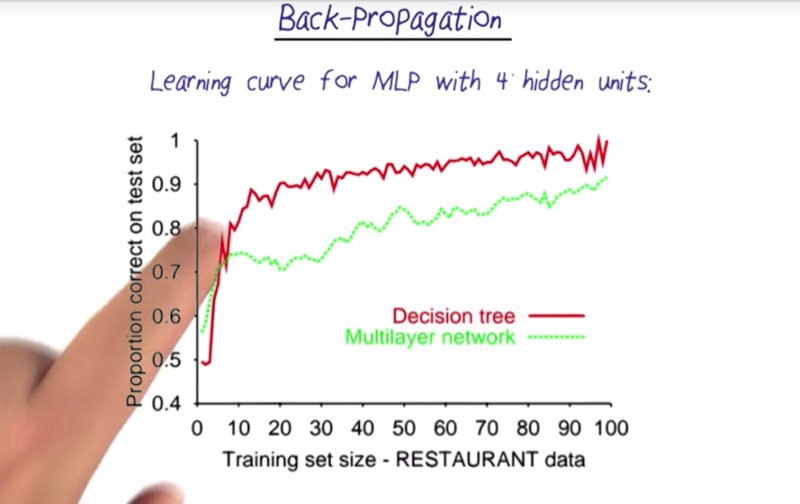## Deep Learning

• neural nets have limitations: need computation power, need more training set but still can be limited on the types of problem it suits.

# Unsupervised Learning

• or classification. The unsupervised learning algorithm classify data into sub-classes and figure out each which class each case fits in.

## k-Means and EM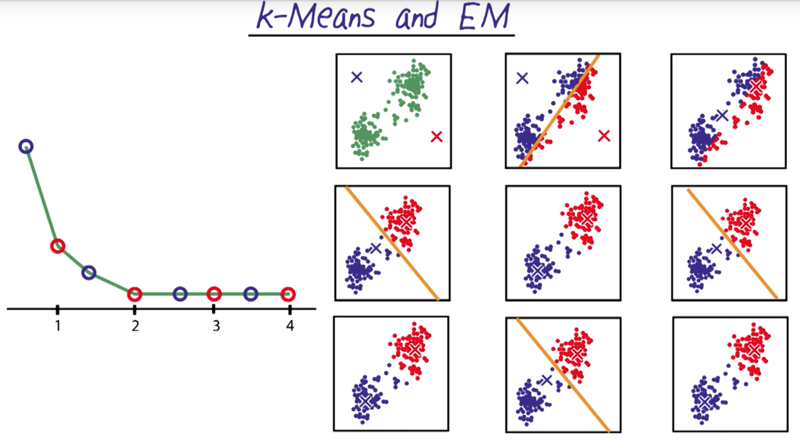• K-means start with randomly initiating the means and generate decision boundaries to separate the data set. the means of the separated data are then recalculated. Then new decision try will be generated to classify the data again. repeat the process until there is no change in the classification anymore.
• this is the expectation-maximization procedure
• for data that is hard to converge or avoid local maxima, random restart technique can be used.

## EM and Mixture of Gaussians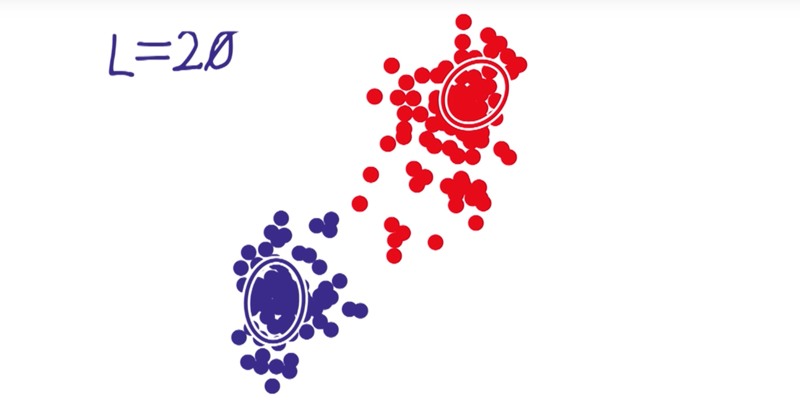• instead of means, we can use k-Gaussians with the EM procedure to do classification.

Readings on EM and Mixture Models

``````20171101 初稿
``````